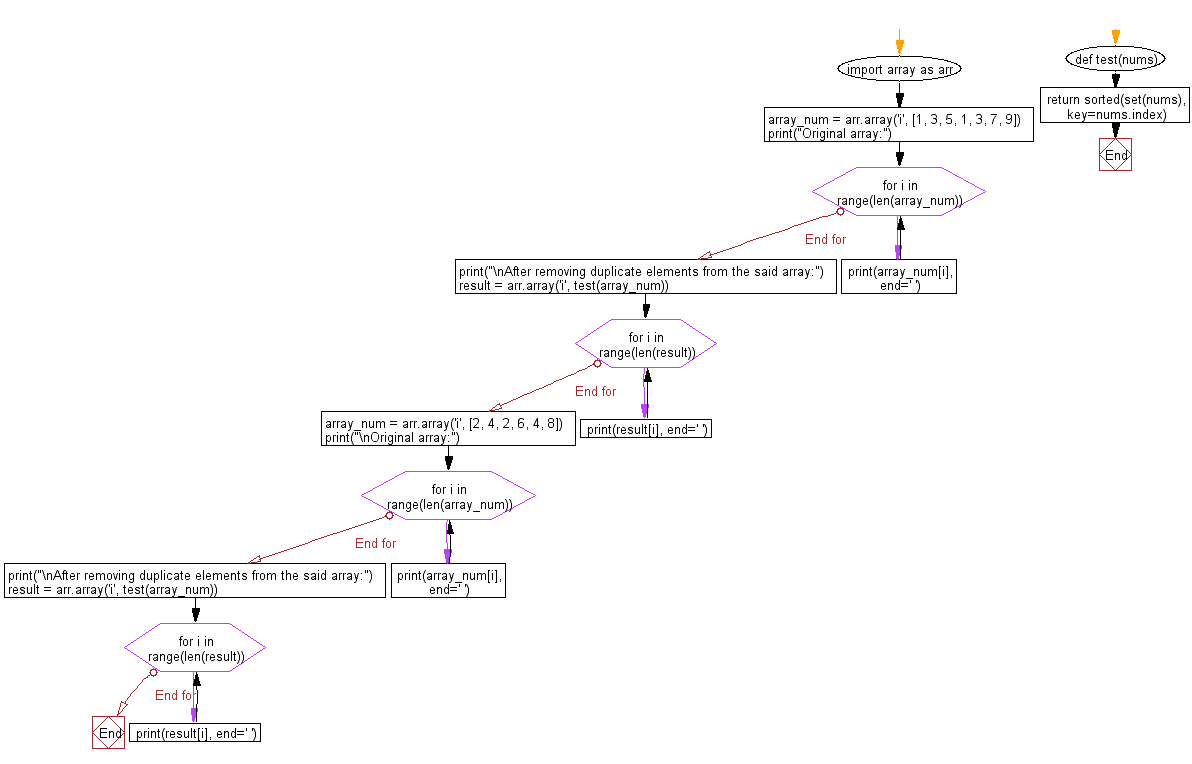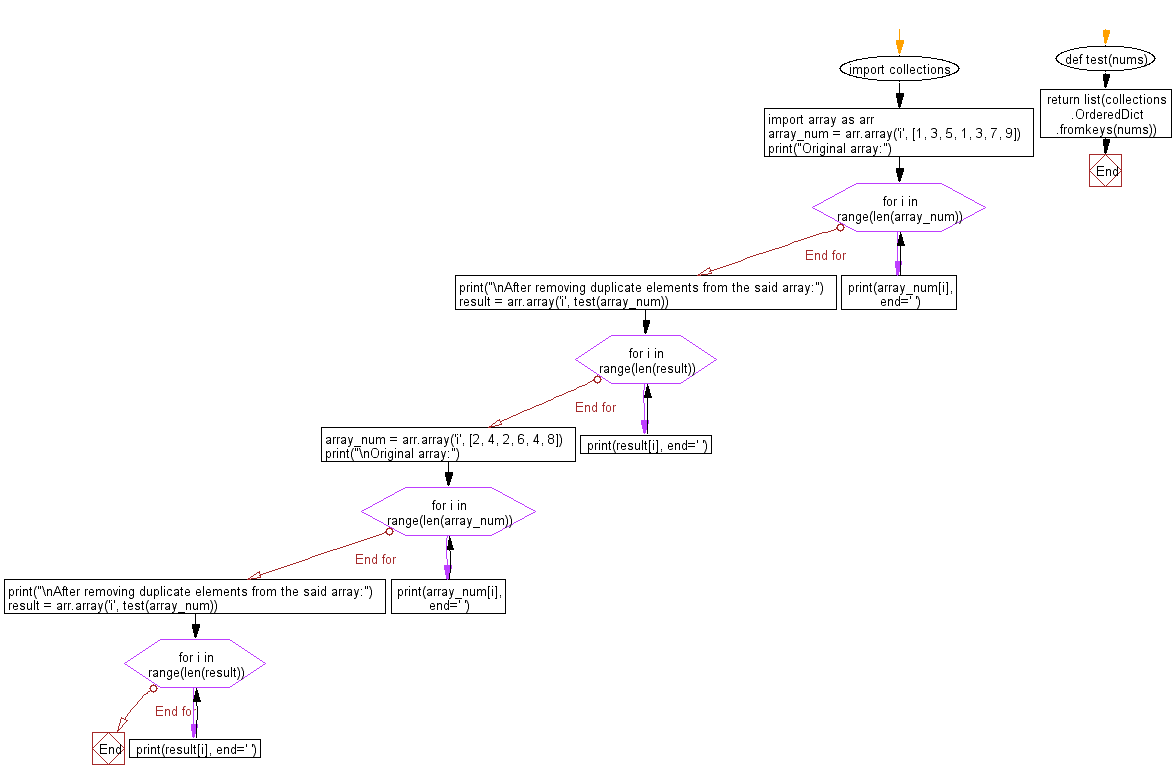﻿ Python: Remove all duplicate elements from a given array - w3resource

# Python: Remove all duplicate elements from a given array

## Python: Array Exercise-23 with Solution

Write a Python program that removes all duplicate elements from an array and returns a new array.

Sample Solution-1:

Python Code:

``````import array as arr
def test(nums):
return sorted(set(nums),key=nums.index)

array_num = arr.array('i', [1, 3, 5, 1, 3, 7, 9])
print("Original array:")
for i in range(len(array_num)):
print(array_num[i], end=' ')
print("\nAfter removing duplicate elements from the said array:")
result = arr.array('i', test(array_num))
for i in range(len(result)):
print(result[i], end=' ')
array_num = arr.array('i', [2, 4, 2, 6, 4, 8])
print("\nOriginal array:")
for i in range(len(array_num)):
print(array_num[i], end=' ')
print("\nAfter removing duplicate elements from the said array:")
result = arr.array('i', test(array_num))
for i in range(len(result)):
print(result[i], end=' ')
```
```

Sample Output:

```Original array:
1 3 5 1 3 7 9
After removing duplicate elements from the said array:
1 3 5 7 9
Original array:
2 4 2 6 4 8
After removing duplicate elements from the said array:
2 4 6 8
```

Flowchart:Sample Solution-2:

Python Code:

``````import collections
import array as arr
def test(nums):
return list(collections.OrderedDict.fromkeys(nums))

array_num = arr.array('i', [1, 3, 5, 1, 3, 7, 9])
print("Original array:")
for i in range(len(array_num)):
print(array_num[i], end=' ')
print("\nAfter removing duplicate elements from the said array:")
result = arr.array('i', test(array_num))
for i in range(len(result)):
print(result[i], end=' ')
array_num = arr.array('i', [2, 4, 2, 6, 4, 8])
print("\nOriginal array:")
for i in range(len(array_num)):
print(array_num[i], end=' ')
print("\nAfter removing duplicate elements from the said array:")
result = arr.array('i', test(array_num))
for i in range(len(result)):
print(result[i], end=' ')
``````

Sample Output:

```Original array:
1 3 5 1 3 7 9
After removing duplicate elements from the said array:
1 3 5 7 9
Original array:
2 4 2 6 4 8
After removing duplicate elements from the said array:
2 4 6 8
```

Flowchart:Python Code Editor: